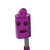# 1. Solution A is sweeter than solution B and thicker than solution E.2. Solution B is sweeter than solution E, thinner

1. Solution A is sweeter than solution B and thicker than solution E.

2. Solution B is sweeter than solution E, thinner than solution D and thicker than solution 3. Solution C is sweeter than solution D and thicker than solution E.

4. Solution D is sweeter than solution B and thinner than solution A.

5. Solution E is the fourth sweetest solution and the thinnest among the five solutions.

If the first four statements are true, then the fifth statement is:​

### 1 thought on “1. Solution A is sweeter than solution B and thicker than solution E.<br /><br />2. Solution B is sweeter than solution E, thinner”

1.Step-by-step explanation:

fifth is

false

bhai